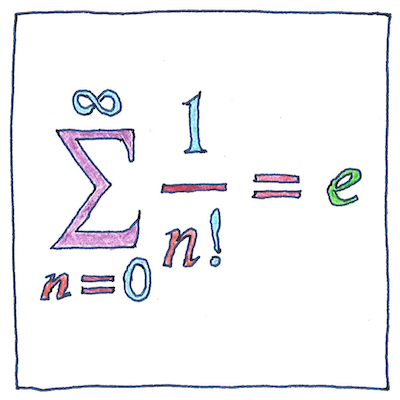# 1683

## The book of science

Tom Sharp

 Jacob Bernoulli, Leonhard Euler mathematics

## Euler’s number

• The first irrational constant was π,
• known as Archimedes’ constant.
• The second was √2,
• known as Pythagoras’ constant.
• The third was e,
• known as Euler’s number.
• Jacob Bernoulli was the first
• to find Euler’s number
• as the result of compounding interest
• when the interval approaches zero,
• known as continuous compounding.
• Leonhard Euler identified e
• as a base for logarithms
• because the number 1
• is common to all counting systems,
• because the logarithm base e of 1 is 1,
• and because the derivative
• of logarithm base e of 1 is 1.

## Transcendental constant

• For something characterized as transcendental,
• it’s odd that e pops up so frequently.
• as the result of continuous compounding
• as the probability of always losing
• in integral expressions for bell curves
• in expressions for the rate of radioactive decay
• in the formula for distances on a Mercator map
• as the area under the hyperbola 1/x
• between 1 and a is the natural log of a.

## Approximation

• Euler’s number is approximately equal to
• 2.718281828459045235360287471352662497757247093699959
• but it is exactly equal to
• the limit as n approaches infinity
• of 1 plus 1 over n to the power of n,
• but go ahead and try to write that out.
• Without being subject
• to the law of diminishing returns,
• you may get as close as you like.

$\underset{\mathit{n\to \infty }}{lim}{\left(1+\frac{1}{n}\right)}^{n}=\sum _{n=0}^{\infty }\frac{1}{\mathit{n}!}=e$

Key to the mathematical symbols:

lim
The limit
n→∞
as n approaches infinity
(1 + 1/n)n
of 1 + the reciprocal of n to the power of n
=
equals
the sum
n = 0
as n goes from zero
to infinity
1/n!
of the reciprocal of n factorial, that is, the inverse of n(n - 1)(n - 2) . . . 1
=
equals
e
the irrational transcendental constant and base of the natural logarithms known as Euler’s number